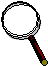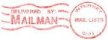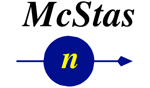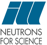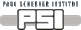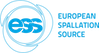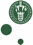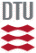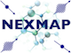McStas: Elliptic_guide_gravity Component

# The `Elliptic_guide_gravity` Component

Perfect elliptic guide which allow for simulations with gravity. The guide mirrors can be divided into segments with individual m-values. Parabolic guide components can also be simulated.

## Identification

• Author: Henrik Bo Hoffmann Carlsen and Mads Bertelsen
• Origin: NBI
• Date: 27 Aug 2012
• Version:(Unknown)

## Description

```
The perfect elliptic guide is centered along the z-axis with the entrance
and exit in the xy-plane. The horizontal and vertical ellipses defining
the guide geometry is by default set by two focal points.
These are placed a distance away from the guide openings along the z-axis;
if distance given is positive, when the focal point is outside the guide.

Multiple options for defining these ellipse exist including approximation of
parabolas and half ellipses (mid point of the ellipse or one of the guide openings)

The guide coating parameters can be set for each side of the guide.
Furthermore the m-value can be specified for user defined segments
of the guide.

Example 1, Elliptical definition using focal points:
Elliptic_guide_gravity(
l=50,
linxw=5,linyh=5,loutxw=10,loutyh=10,
xwidth=0.05,yheight=0.05,
R0=0.99,Qc=0.0219,alpha=6.07,m=1.0,W=0.003
)

Example 2: Half elliptical definition:
Elliptic_guide_gravity(
l=50,
linxw=5,linyh=5,loutxw=10,loutyh=10,
xwidth=0.1,yheight=0.1,
R0=0.99,Qc=0.0219,alpha=6.07,m=1.0,W=0.003,
option = "halfEllipse",
dimensionsAt = "entrance"
)

Example 3: Parabolic approximation:
Elliptic_guide_gravity(
l=50,
linxw=5,linyh=5,loutxw=1e6,loutyh=1e6, // values larger than 1e8 may cause erroneous results
xwidth=0.1,yheight=0.1,
R0 = 0.99,Qc=0.0219,alpha=6.07,m=1.0,W=0.003,
dimensionsAt = "exit"
)

Example 4: Elliptical definition with varying m-values:
Elliptic_guide_gravity(
l=50,
linxw=5,linyh=5,loutxw=10,loutyh=10,
xwidth=0.1,yheight=0.1,
R0 = 0.99,Qc=0.0219,alpha=6.07,m=1.0,W=0.003,
mvaluesright=marray,mvaluesleft=marray,mvaluestop=marray,mvaluesbottom=marray
)
where marray is initialized as
for(iter=0; iter < 50; iter++){ marray[iter] = 2; }
And Declared as
double mValues;

```
WARNING: This is a contributed Component.

## Input parameters

Parameters in boldface are required; the others are optional.
Name Unit Description Default
mvaluesright pointer Pointer to array of m-values, right mirror NULL
mvaluesleft pointer - same, left mirror NULL
mvaluestop pointer - same, top mirror NULL
mvaluesbottom pointer - same, bottom mirror NULL
seglength pointer Pointer to array of segment lengths for discrete mirror description NULL
l m length of the guide
xwidth see dimensionsAt [m] width at the guide entry, mid or exit 0
yheight see dimensionsAt [m] height at the guide entry, mid or exit 0
linxw m distance from 1st focal point to guide entrance - left and right horizontal mirrors 0
loutxw m distance from 2nd focal point to guide exit - left and right horizontal mirrors 0
linyh m distance from 1st focal point to guide entrance - top and bottom vertical mirrors 0
loutyh m distance from 2nd focal point to guide exit - top and bottom vertical mirrors 0
majorAxisxw m direct defination of the guide geometry, will ignore w,h lin and lout parameters if this is nonzero. Length of the axis parallel to the z for the horizontal ellipse 0
minorAxisxw m direct defination of the guide geometry, will ignore w,h lin and lout parameters if this is nonzero. Length of the axis Perpendicular to the z for the horizontal ellipse 0
majorAxisyh m direct defination of the guide geometry, will ignore w,h lin and lout parameters if this is nonzero. Length of the axis parallel to the z for the vertical ellipse 0
minorAxisyh m direct defination of the guide geometry, will ignore w,h lin and lout parameters if this is nonzero. Length of the axis Perpendicular to the z for the vertical ellipse 0
majorAxisoffsetxw m direct defination of the guide geometry, distance between the center of the horizontal ellipse and the guide entrance 0
majorAxisoffsetyh m direct defination of the guide geometry, distance between the center of the vertical ellipse and the guide entrance 0
dimensionsAt string define whether xwidth and yheight sets the size of he opening, minor axis or the end of the guide. "entrance"
option string options are 'ellipse' and 'halfEllipse'. Ellipse is defined by both the focal points, while halfEllipse locked the center of the ellipse either the entrance or exit of the guide, and use the focal point of the other end to define the ellipse "ellipse"
R0 1 Low-angle reflectivity 0.99
Qc AA-1 Critical scattering vector 0.0218
alpha AA Slope of reflectivity 6.07
m 1 m-value of material for all mirrors, zero means complete absorption. 2
W AA-1 Width of supermirror cut-off 0.003
alpharight AA Slope of reflectivity for right vertical mirror -1
mright 1 m-value of material for right vertical mirror -1
alphaleft AA Slope of reflectivity for left vertical mirror -1
mleft 1 m-value of material for left vertical mirror -1
alphatop AA Slope of reflectivity for top horizontal mirror, overwrites alpha -1
mtop 1 m-value of material for top horizontal mirror, overwrites m -1
alphabottom AA Slope of reflectivity for bottom horizontal mirror -1
mbottom 1 m-value of material for bottom horizontal mirror -1
verbose bool Give extra information about calculations "on"
curvature m Simulate horizontal radius of curvature by centripetal force added to the gravity. Note: Does not curve the guide in mcdisplay but "curves the neutron". Has opposite sign definition of Guide_curved. 0

## Output parameters

Name Unit Description Default
guideInfo
latestParticleCollision
Gx
Gy
Gz
Gx0
Gy0
Gz0
Circ
dynamicalSegLength

## Links

• Source code for `Elliptic_guide_gravity.comp`.

Generated automatically by McDoc, Peter Willendrup <peter.willendrup@risoe.dk> / Tue Apr 2 12:43:09 2019

Last Modified: Tuesday, 02-Apr-2019 14:43:09 CEST